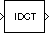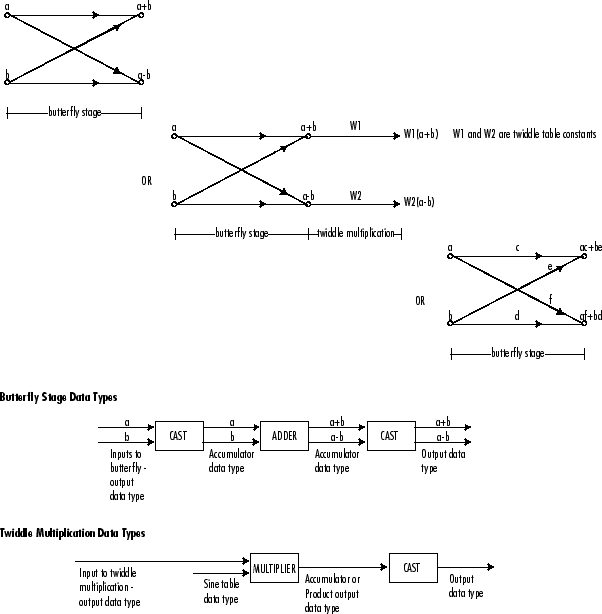# IDCT

Inverse discrete cosine transform (IDCT) of input

## Library

Transforms

`dspxfrm3`

•## Description

The IDCT block computes the inverse discrete cosine transform (IDCT) of each channel in the M-by-N input matrix, `u`.

`y = idct(u) % Equivalent MATLAB code `

For all N-D input arrays, the block computes the IDCT across the first dimension. The size of the first dimension (frame size), must be a power of two. To work with other frame sizes, use the Pad block to pad or truncate the frame size to a power-of-two length.

When the input is an M-by-N matrix, the block treats each input column as an independent channel containing M consecutive samples. The block outputs an M-by-N matrix whose lth column contains the length-M IDCT of the corresponding input column.

`$y\left(m,l\right)=\sum _{k=1}^{M}w\left(k\right)u\left(k,l\right)\mathrm{cos}\frac{\pi \left(2m-1\right)\left(k-1\right)}{2M},\begin{array}{ccc}& & m=1,...,M\end{array}$`

where

`$w\left(k\right)=\left\{\begin{array}{l}\frac{1}{\sqrt{M}}\\ \sqrt{\frac{2}{M}}\end{array}\begin{array}{c}\begin{array}{l}\\ ,\\ \\ ,\end{array}\\ \end{array}\begin{array}{ll}\hfill & \hfill \\ \hfill & k=1\hfill \\ \hfill & \hfill \\ \hfill & 2\le k\le M\hfill \\ \hfill & \hfill \end{array}$`

The Sine and cosine computation parameter determines how the block computes the necessary sine and cosine values. This parameter has two settings, each with its advantages and disadvantages, as described in the following table.

Sine and Cosine Computation Parameter SettingSine and Cosine Computation MethodEffect on Block Performance

`Table lookup`

The block computes and stores the trigonometric values before the simulation starts, and retrieves them during the simulation. When you generate code from the block, the processor running the generated code stores the trigonometric values computed by the block in a speed-optimized table, and retrieves the values during code execution.

The block usually runs much more quickly, but requires extra memory for storing the precomputed trigonometric values.

`Trigonometric fcn`

The block computes sine and cosine values during the simulation. When you generate code from the block, the processor running the generated code computes the sine and cosine values while the code runs.

The block usually runs more slowly, but does not need extra data memory. For code generation, the block requires a support library to emulate the trigonometric functions, increasing the size of the generated code.

### Fixed-Point Data Types

The following diagrams show the data types used within the IDCT block for fixed-point signals. You can set the sine table, accumulator, product output, and output data types displayed in the diagrams in the IDCT block dialog as discussed in Parameters.

Inputs to the IDCT block are first cast to the output data type and stored in the output buffer. Each butterfly stage processes signals in the accumulator data type, with the final output of the butterfly being cast back into the output data type.The output of the multiplier is in the product output data type when at least one of the inputs to the multiplier is real. When both of the inputs to the multiplier are complex, the result of the multiplication is in the accumulator data type. For details on the complex multiplication performed, see Multiplication Data Types.

Note

When the block input is fixed point, all internal data types are signed fixed point.

## Parameters

Main Tab

Sine and cosine computation

Sets the block to compute sines and cosines by either looking up sine and cosine values in a speed-optimized table (`Table lookup`), or by making sine and cosine function calls (`Trigonometric fcn`). See the table in the Description section.

Data Types Tab

Rounding mode

Select the rounding mode for fixed-point operations. The sine table values do not obey this parameter; they always round to `Nearest`.

Saturate on integer overflow

When you select this parameter, the block saturates the result of its fixed-point operation. When you clear this parameter, the block wraps the result of its fixed-point operation. For details on `saturate` and `wrap`, see overflow mode for fixed-point operations.

Note

The Rounding mode and Saturate on integer overflow parameters have no effect on numeric results when all these conditions are met:

• Product output data type is ```Inherit: Inherit via internal rule```.

• Accumulator data type is ```Inherit: Inherit via internal rule```.

With these data type settings, the block operates in full-precision mode.

Sine table

Choose how you specify the word length of the values of the sine table. The fraction length of the sine table values always equals the word length minus one. You can set this parameter to:

• A rule that inherits a data type, for example, ```Inherit: Same word length as input```

• An expression that evaluates to a valid data type, for example, `fixdt(1,16)`

The sine table values do not obey the Rounding mode and Saturate on integer overflow parameters; instead, they are always saturated and rounded to `Nearest`.

Product output

Specify the product output data type. See Fixed-Point Data Types and Multiplication Data Types for illustrations depicting the use of the product output data type in this block. You can set this parameter to:

• A rule that inherits a data type, for example, ```Inherit: Inherit via internal rule```. For more information on this rule, see Inherit via Internal Rule.

• An expression that evaluates to a valid data type, for example, `fixdt(1,16,0)`

Click the buttonto display the Data Type Assistant, which helps you set the Product output parameter.

Accumulator

Specify the accumulator data type. See Fixed-Point Data Types for illustrations depicting the use of the accumulator data type in this block. You can set this parameter to:

• A rule that inherits a data type, for example, ```Inherit: Inherit via internal rule```. For more information on this rule, see Inherit via Internal Rule.

• An expression that evaluates to a valid data type, for example, `fixdt(1,16,0)`

Click the buttonto display the Data Type Assistant, which helps you set the Accumulator parameter.

Output

Specify the output data type. See Fixed-Point Data Types for illustrations depicting the use of the output data type in this block. You can set this parameter to:

• A rule that inherits a data type, for example, ```Inherit: Inherit via internal rule```.

When you select `Inherit: Inherit via internal rule`, the block calculates the output word length and fraction length automatically. The internal rule first calculates an ideal output word length and fraction length using the following equations:

`$W{L}_{idealoutput}=W{L}_{input}+floor\left({\mathrm{log}}_{2}\left(DCTlength-1\right)\right)+1$`
`$F{L}_{idealoutput}=F{L}_{input}$`

Using these ideal results, the internal rule then selects word lengths and fraction lengths that are appropriate for your hardware. For more information, see Inherit via Internal Rule.

• An expression that evaluates to a valid data type, for example, `fixdt(1,16,0)`

Click the buttonto display the Data Type Assistant, which helps you set the Output parameter.

Output Minimum

Specify the minimum value that the block should output. The default value is `[]` (unspecified). Simulink® software uses this value to perform:

• Simulation range checking (see Specify Signal Ranges (Simulink))

• Automatic scaling of fixed-point data types

Output Maximum

Specify the maximum value that the block should output. The default value is `[]` (unspecified). Simulink software uses this value to perform:

• Simulation range checking (see Specify Signal Ranges (Simulink))

• Automatic scaling of fixed-point data types

Lock data type settings against changes by the fixed-point tools

Select this parameter to prevent the fixed-point tools from overriding the data types you specify on the block mask.

## Supported Data Types

PortSupported Data Types

Input

• Double-precision floating point

• Single-precision floating point

• Fixed point (signed and unsigned)

• 8-, 16-, and 32-bit signed integers

• 8-, 16-, and 32-bit unsigned integers

Output

• Double-precision floating point

• Single-precision floating point

• Fixed point (signed only)

• 8-, 16-, and 32-bit signed integers

## Extended Capabilities

### Blocks

Introduced before R2006a

Watch now